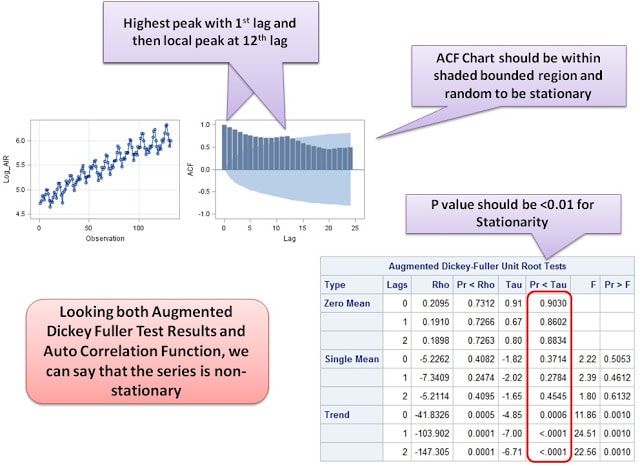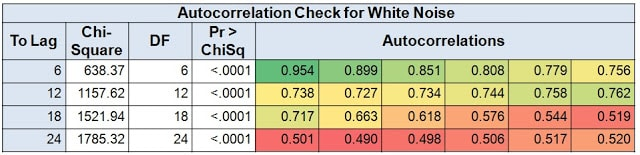# Time Series Forecasting - ARIMA [Part 2]

In this part we would cover the process of performing ARIMA with SAS and a little theory in between.

Hope you have gone through the Part-1 of this series, here comes the Part-2 .

Data File Location :
Library - SASHELP
Data set - AIR
Data Preparation Steps For ARIMA Modeling

1. Check if there is variance that changes with time - Volatility. For ARIMA, the volatility should not be very high.
2. If the volatility is very high, we need to make it non-volatile.
3. Check for Stationary - a series should be stationary before performing ARIMA.
4. If data is non-stationary, we need to make it stationary.
5. Check for Seasonality in the data

Step 1 : Check the series

As a matter of practice, we first plot the time series and have a cursory look upon it. It can be done directly in SAS using following code :
proc sgplot data = sashelp.AIR;
series x = date Y = AIR;
run;
quit;
It would give you the following plot in the result window :SAS : Time Series Modeling

It is clear from the chart above that the series of AIR is having an increasing trend and consistent pattern over time. The peaks are at a constant time interval which is indicative of presence of seasonality in the series.
This is a non-stationary series for sure and hence we need to make it stationary first.
Practically, ARIMA works well in case of such types of series with a clear trend and seasonality. We first separate and capture the trend and seasonality component off the time-series and we are left with a series i.e. stationary. This stationary series is forecasted using ARIMA and then final forecasting incorporates the pre-captured trend and seasonality.

We would understand it in details further  in Step-3.

Step 2 : Check the volatility of the series

Volatility is the degree of variation of a time-series over time. For ARIMA, the volatility should not be very high. For checking the volatility of time-series, we do a scatter plot using the following SAS code :
Proc gplot data=SAShelp.AIR;
plot Date * AIR;
Run;Quit;
It would give you the following plot in the result window :Check the volatility of Series
The highlighted area is showing the diverging pattern (Fan shaped) of the  scatter plot and hence depicting that the data is volatile. Ideally, the highlighted pattern should be parallel for ARIMA modeling.

Step 3 : Treatment of Volatile Series

We need to make the series non-volatile and move ahead. We would transform the AIR series and remove volatility. Generally a hit and trail method for transformation is used, but we would suggest to not to waste your time.

Box-Cox Transformation can be used to help you out and recommend the suitable transformation.
Proc Transreg Data = sashelp.AIR;
Model BOXCOX (AIR) = Identity(Date);
Run;

You get following plot along with Lamba value, which is "0" in this case.

Now based on this Lambda value, you can decide the transformation. Take help from the table provided below.Box cox Transformation
In our case, it is suggesting a log transformation, so we do the same. In a new data (Masterdata) we create a new variable (Log_AIR).
Data Masterdata;
Set  SAShelp.AIR;
Log_AIR = log(AIR);Run;
We can check the volatility again of the transformed series, just to be sure, using scatter plot as elaborated above.

Step 4 : Check For Non-Stationarity

Now on the transformed series, we check whether the series is stationary or non-stationary.

For performing ARIMA , a series should be stationary, however if the series is non-stationary, we make it stationary (For more explanation on stationarity, read Part 1 of this series).

Rather than identifying the series's stationarity visually as we have done in step 1, we now use Augmented Dickey-Fuller Unit Ratio Test for the same.

Unit Root - Homogeneous Non-Stationarity Data

Dickey-Fuller test
The Dickey-Fuller test is used to test the null hypothesis that the time series exhibits a lag d unit root against the alternative of stationarity.
Null Hypothesis : Non-Stationary
Alternative Hypothesis : Stationary

There are three types by which you can calculate test statistics of dickey-fuller test.

1. Zero Mean - No Intercept. Series is a random walk without drift.
2. Single Mean - Includes Intercept. Series is a random walk with drift.
3. Trend - Includes Intercept and Trend. Series is a random walk with linear trend.
All the above test statistics are computed from the OLS regression model.

Uncertainty about what test version to use, i.e. about including the intercept and time trend terms.
Inappropriate exclusion or inclusion of these terms substantially affects test reliability.
Using of prior knowledge (for instance, as result of visual inspection of a given time series) about whether the intercept and time trend should be included is the mostly recommended way to overcome the difficulty mentioned.
We run Proc ARIMA with Stationarity = (ADF) option to do so :
PROC ARIMA DATA= Masterdata ;
IDENTIFY VAR = log_Air STATIONARITY= (ADF) ;
RUN;
QUIT;
There are many outputs of the above code, a part of which is used for checking stationarity:ARIMA : Check Stationary
Important Note :
Check Tau Statistics (Pr < Tau) in ADF Unit Root Tests table. It should be less than 0.05 to say data is stationary at 5% level of significance.

Step 5 : Make Non-Stationary Data Stationary

Post establishing the non-stationarity of the series, we need to make the series stationary. Differencing process is used for making the series stationary.
Differencing : Transformation of the series to a new time series where the values are the differences between consecutive values
Differencing Procedure may be applied consecutively more than once, giving rise to the "first differences", "second differences", etc.

Differencing Orders :
1st order     :  xt = xt – xt-1. For eg. Sales - lag1(Sales)
2nd order  : 2xt = (xt - xt-1 )=xt – 2xt-1 + xt-2
It is unlikely that more than two differencing orders would ever be required.
Note :  If there is a physical explanation for a trend or seasonal cycle : use regression to make series stationary.

For that we use the output of the Step-3 code itself. While we have run the code above, we have got "Autocorrelation Check for White Noise" along with " Augmented Dickey-Fuller Unit Root Tests".

Looking at "Autocorrelation Check for White Noise", we decide the order(s) of differencing required.Stationary : Order of Differencing
A heat map has been made using Excel for demonstration, SAS output is black and white only.

The first row of the above autocorrelation matrix shows correlation of time-series with 1st to 6th lags, second row show the same for 7th to 12th lags...and so on ... The same is visible in ACF chart provided in Step-3 visuals.
We can see that in above matrix the highest auto-correlation exists with 1st lag, it starts decreasing but again increases to attain a local peak at 12th lag.

Step 6 : Check Seasonality

Highest Correlation with 1st Lag indicates towards the presence of trend and that with 12th lag indicates an annual seasonality. Hence we need to do differencing at first and Twelfths orders.

We perform differencing and check the stationarity again.
PROC ARIMA DATA= masterdata ;
IDENTIFY VAR = Log_Air (1,12) STATIONARITY= (ADF) ;
RUN;quit;
We have used 1 and 12 in bracket to define the 1st and 12th order of differencing.

Check whether data is stationary
Check Tau Statistics (Pr < Tau) in ADF Unit Root Tests table again and see if the value <0.05 to say data is stationary at 5% level of significance.

How this differencing actually worked :

1. First order (1) Differencing removes the trend, but Seasonality still exists.

2. Second Order (12) Differencing removes the seasonality.

How to do it with MS Excel:
First subtract first lag from each observation and plot it. Then in this new series subtract 12th lag from each observation.

Step 7 : Split Data into Training and Validation

Now we can break the data into Training and Validation samples.We cannot use random sampling like we do in regression models to split the data. Instead, we can use recent data for validation and remaining data be used to train the model. We would develop ARIMA model and forecast on Testing part and would check the results on Validation part.
Data Training Validation;
Set Masterdata;
If date >= '01Jan1960'd then output Validation;
Else output Training;
Run;
After these 7 steps, we would train ARIMA model on Training Dataset in Part 3 of this series.

Check out : Time Series Forecasting - ARIMA [Part 3]

This article was originally written by Rajat Agarwal, later Deepanshu gave final touch to the post. Rajat is an analytics professional with more than 8 years of work experience in diverse business domains. He has gained expert knowledge in Excel and SAS. He loves to create innovative and imaginative dashboards with Excel. He is founder and lead author cum editor at Ask Analytics.Share
Related Posts
1 Response to "Time Series Forecasting - ARIMA [Part 2]"
1.despite doing everything - using MINIC, my autocorrelation is still significant, what should I do

Autocorrelation Check of Residuals

To Chi- Pr >
Lag Square DF ChiSq --------------------Autocorrelations--------------------

6 19.46 4 0.0006 -0.045 -0.094 0.282 -0.178 0.079 0.279
12 41.37 10 <.0001 -0.260 0.024 0.311 -0.199 0.005 -0.121
18 64.20 16 <.0001 -0.275 0.212 -0.075 -0.193 0.207 -0.072
24 92.59 22 <.0001 -0.125 0.151 -0.180 -0.168 0.272 -0.250

Next → ← Prev